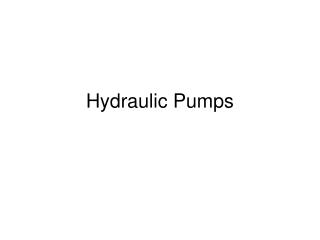DownloadDownload PresentationHydraulic Pumps

# Hydraulic Pumps

Download Presentation## Hydraulic Pumps

- - - - - - - - - - - - - - - - - - - - - - - - - - - E N D - - - - - - - - - - - - - - - - - - - - - - - - - - -
##### Presentation Transcript

1. Hydraulic Pumps

2. Pump Objectives: • 1. Explain the difference in Positive and Non-positive displacement pumps. • 2. Explain the difference in Fixed displacement and variable displacement pumps. • 3. Describe the three major types of pumps. • 4. Explain the difference in the two types of gear pumps. • 5. Explain the difference in the two types of vane pumps. • 6. Describe the difference in the two major types of piston pumps. • 7. List the ways in which pumps are sized. • 8. List possible sources of problems with pumps

3. Sizing and Selecting the Right Pump • Main factors • Fixed or variable • Pressure psi (Max) 100 to 4000 psi • Horsepower (HP) • HP = (GPM x system pressure in PSI) / 1714 x % Pump efficiency • HP + GPM x PSI X .000583 • Displacement or volume (cubic inches– GPM) • GPM = (Cubic Inches / minute) / 231 Ex. 462 GPM / 231 = 2 • These are both a function of surface area in pump • However displacement can be affect by input speed of pump • Other factors to consider • RPM (1800 or 3600) • Direction of rotation (CCW) • Inlet or outlet size • Mounting • Shaft size

4. Pump Efficiency Actual output (GPM) ------------------Theoretical Output (GPM) • Volumetric Efficiency = • Example: • Theoretical = 10 GPM, actual = 9 GPM • What is the volumetric efficiency? 9--- = .9 x100 = 90 % 10

5. Example 2 • For example, a hydraulic pump with a • theoretical output of 100 GPM, and • an actual output of 94 GPM • at 5000 PSI and 120 SUS • is said to have a volumetric efficiency of 94% at 5000 PSI and 120 SUS.

6. What is the significance of volumetric efficiency? • As a hydraulic pump wears in service, internal leakage increases and therefore the percentage of theoretical flow available to do useful work (volumetric efficiency) decreases. If volumetric efficiency falls below a level considered acceptable for the application, the pump will need to be overhauled.

7. Pump Efficiency • Mechanic Efficiency is the Overall Efficiency compared to Volumetric Efficiency …. Difference due to wear and friction • Overall Efficiency is the ratio of power output to mechanical power input of the pump (HP input compared to HP output)

8. Pump Problems • Too much pressure rated at 2000 operated at something over that for long periods of time. • Heats the fluid • Wears bearing • Wear • Contaminates – Causing wear • Where do they come from? • External -- How do they enter? Loose lines, rusty cylinder with worn wiper seal, fill cap, • Internal –wearing of parts, bad fluids (wrong viscosity—wrong type), heat,

9. Pump Problems • How does contamination affect a hydraulic pump? • Particle contamination accelerates wear of hydraulic components. The rate at which damage occurs is dependent on the internal clearance of the components within the system, the size and quantity of particles present in the fluid, and system pressure. • Particles larger than the component's internal clearances are not necessarily dangerous. Particles the same size as the internal clearances cause damage through friction. However, the most dangerous particles in the long term are those that are smaller than the component's internal clearances. • Particles smaller than 5 microns are highly abrasive. If present in sufficient quantities, these invisible 'silt' particles cause rapid wear, destroying hydraulic pumps and other components.

10. Pump Problems • Fluids – lack of fluid, wrong fluid type, wrong viscosity, hot fluid, cold fluid • Cavitation • Seal failure

11. Pump comparison…. Gear, Vane and Piston • See text…